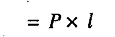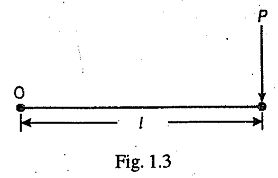### Moment of a Force

Moment of a Force is the turning effect produced by a force, on the body, on which it acts. The moment of a force is equal to the product of the force and the perpendicular distance of the point, about which the moment is required and the line of action of the force. Mathematically, the moment of a force P about point O as shown in Fig 1.3,The unit of moment depends upon the units of force and perpendicular distance. If the force is in newtons and the perpendicular distance in meters, then the unit of moment will be Newton-metre (briefly written as N-m).